# Angle Bisector Theorem

Angle Bisector Theorem
Go back to  'Triangles'

"The kite rises highest against the wind, not with it."

Isn't this an inspiring thought?

Yes, indeed!

Let's have a closer look at the kite. Are you able to see angles being formed at each of its corners?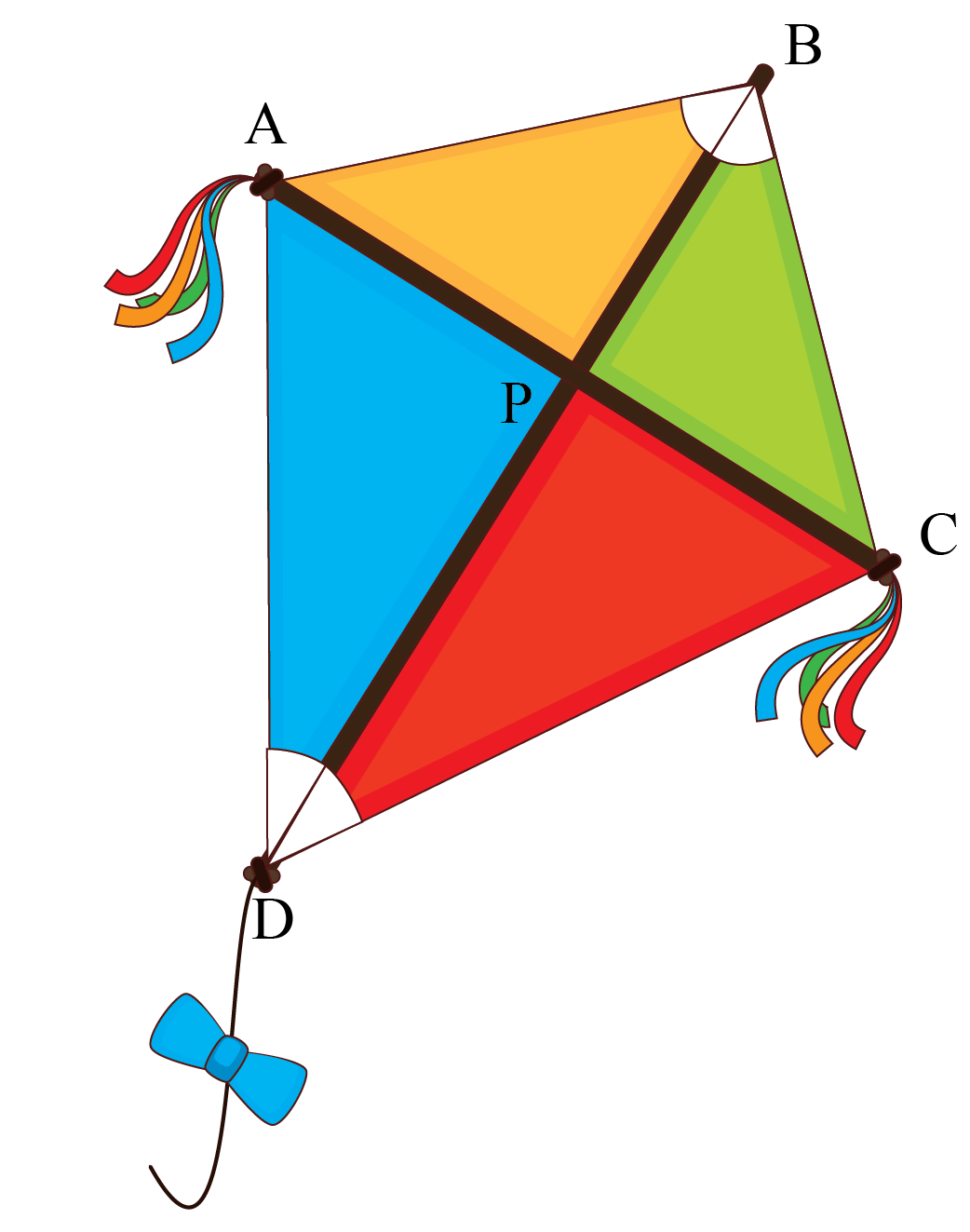In the figure, we can observe that segment BD has divided $$\angle B$$ and  $$\angle D$$ into equal parts.

That's called an angle bisector.

In this short lesson, we will be focusing more on the most important theorem on the angle bisector. To be more precise, we will be learning about the angle bisector theorem proof, angle bisector theorem examples, triangle angle bisector theorem, how to construct angle bisector, and other interesting properties and facts around angle bisectors.

## What Is Angle Bisector Theorem?

Triangle angle bisector theorem states that "In a triangle, the angle bisector of any angle will divide the opposite side in the ratio of the sides containing the angle".

Consider the figure below.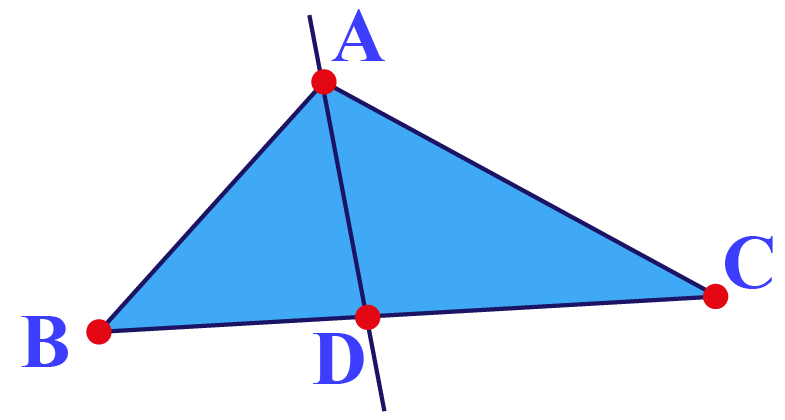Here, AD is the bisector of $$\angle A$$.

According to the angle bisector theorem, $$\dfrac{BD}{DC}=\dfrac{AB}{AC}$$.

### Construction Of Angle Bisector

Use the simulation below to visualize the steps of construction for angle bisector.

## Proof Of Angle Bisector Theorem

### Angle Bisector Theorem Proof

To prove the angle bisector theorem, we will do construction.

Draw a ray CX parallel to AD, and extend BA to intersect this ray at E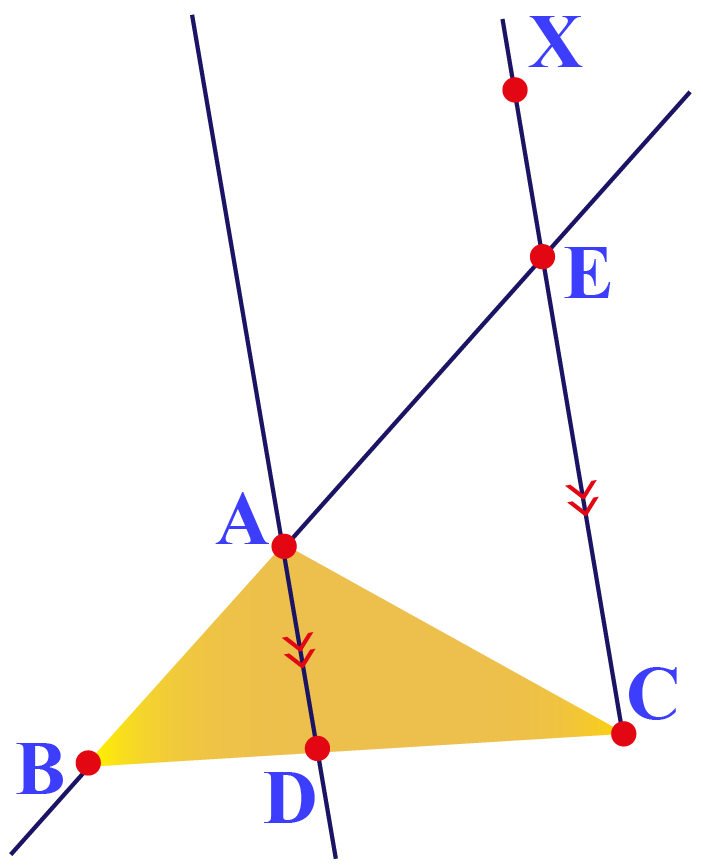By the Basic Proportionality Theorem, we have that if a line is drawn parallel to one side of a triangle to intersect the other two sides in distinct points, the other two sides are divided in the same ratio.

In $$\Delta CBE$$, DA is parallel to CE.

$$\dfrac{BD}{DC}=\dfrac{BA}{AE}\;\;\;\;\;\; \cdots (1)$$

Now, we are left with proving that AE = AC.

Let's mark the angles in the above figure.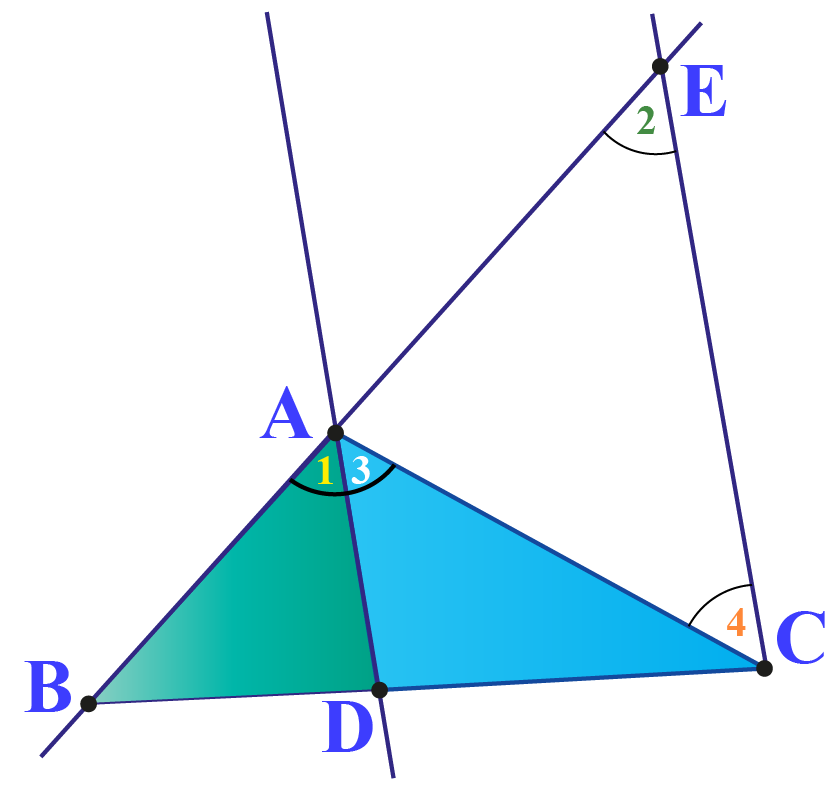Since DA is parallel to CE, we have

1. $$\angle 1=\angle 2$$ (corresponding angles)
2. $$\angle 3=\angle 4$$ (alternate interior angles)

Since AD is the bisector of $$\angle BAC$$, we have $$\angle 1=\angle 3$$.

So, we can say that $$\angle 2=\angle 4$$.

Since sides opposite to equal angles are equal, AC = AE.

Substitute AC for AE in Equation (1).

$$\dfrac{BD}{DC}=\dfrac{BA}{AC}$$

Hence proved.

## Solved Examples

 Example 1

Amy drew a triangle on the board.

She asks if $$\dfrac{AB}{AC}=\dfrac{BD}{DC}$$.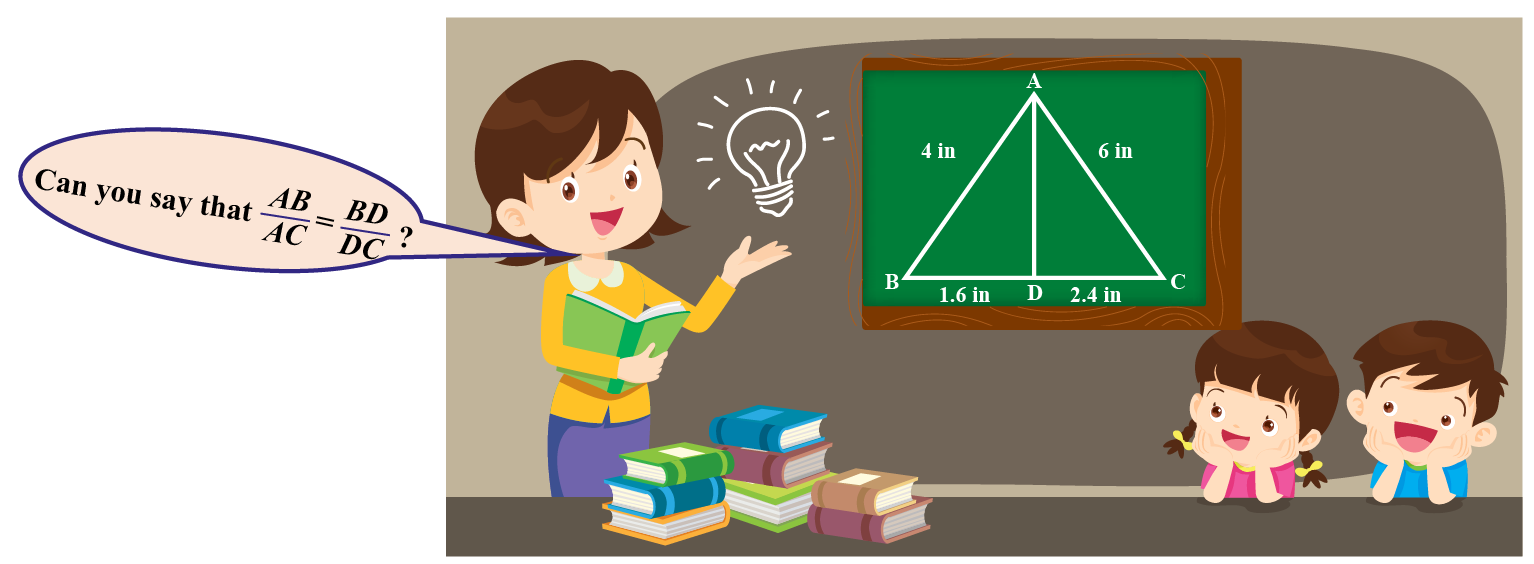Can you answer this?

### Solution

Let's find the ratio $$\dfrac{AB}{AC}$$.

\begin{align}\dfrac{AB}{AC}=\dfrac{4}{6}=\dfrac{2}{3}\end{align}

Let's find the ratio $$\dfrac{BD}{DC}$$.

\begin{align}\dfrac{BD}{DC}=\dfrac{1.6}{2.4}=\dfrac{2}{3}\end{align}

 $$\therefore$$ Both the ratios are equal.
 Example 2

In $$\Delta XYZ$$, XE is the bisector of $$\angle X$$.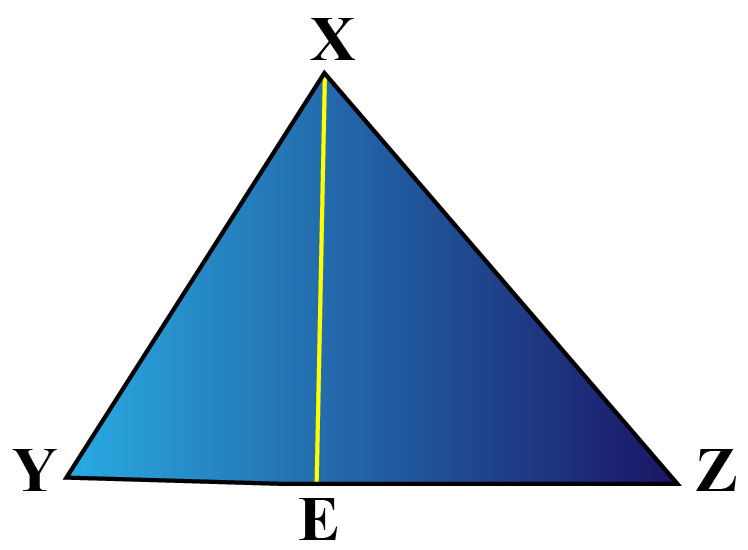Let XY = 4 units, YE = 2 units, and EZ = 3 units.

Can you find the length of XZ?

### Solution

Given that, XE is the bisector of $$\angle X$$.

So, we can use the angle bisector theorem.

According to the question,

\begin{align}\dfrac{YE}{EZ}&=\dfrac{XY}{XZ}\\\dfrac{2}{3}&=\dfrac{4}{XZ}\\XZ&=\dfrac{4}{2}\times 3\\XY&=6\end{align}

 $$\therefore$$ The length of XZ is 6 units.
 Example 3

Look at $$\Delta ABC$$ shown below.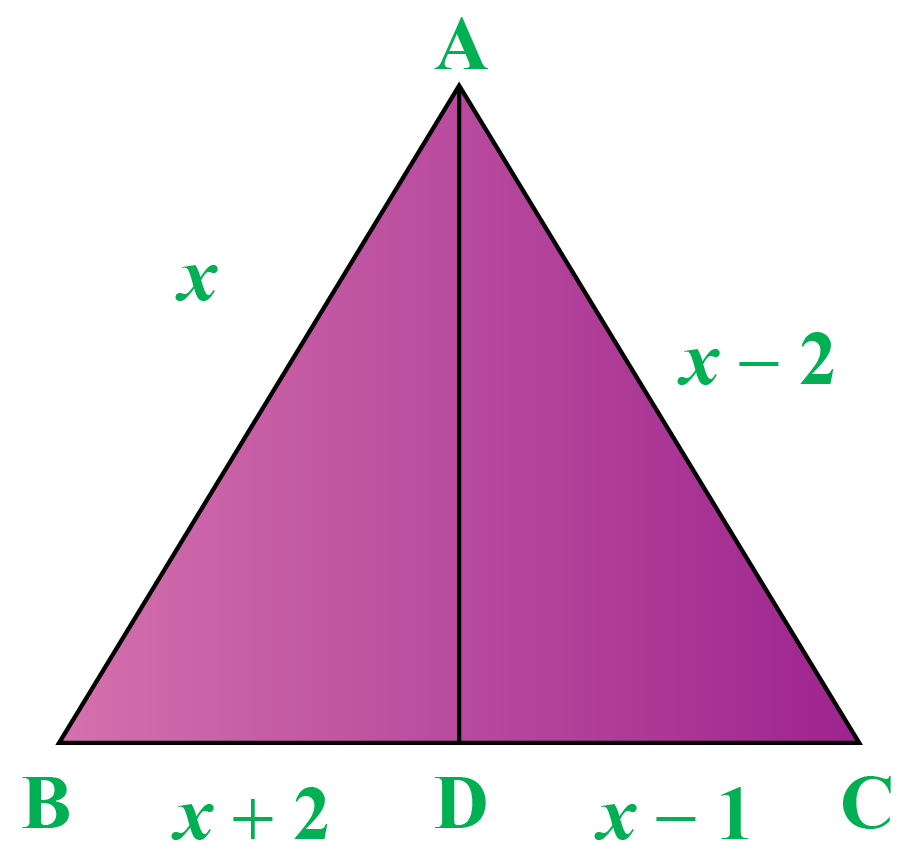If AD bisects $$\angle A$$, can you find the value of $$x$$?

Solution

Given that, AD is the bisector of $$\angle A$$.

So, we can use the angle bisector theorem.

According to the question,

\begin{align}\dfrac{AB}{AC}&=\dfrac{BD}{DC}\\\dfrac{x}{x-2}&=\dfrac{x+2}{x+1}\\x(x-1)&=(x-2)(x+2)\\x^2-x&=x^2-4\\-x&=-4\\x&=4\end{align}

 $$\therefore$$ The value of $$x$$ is 4.
 Example 4

Jenny drew a right-angled triangle as shown.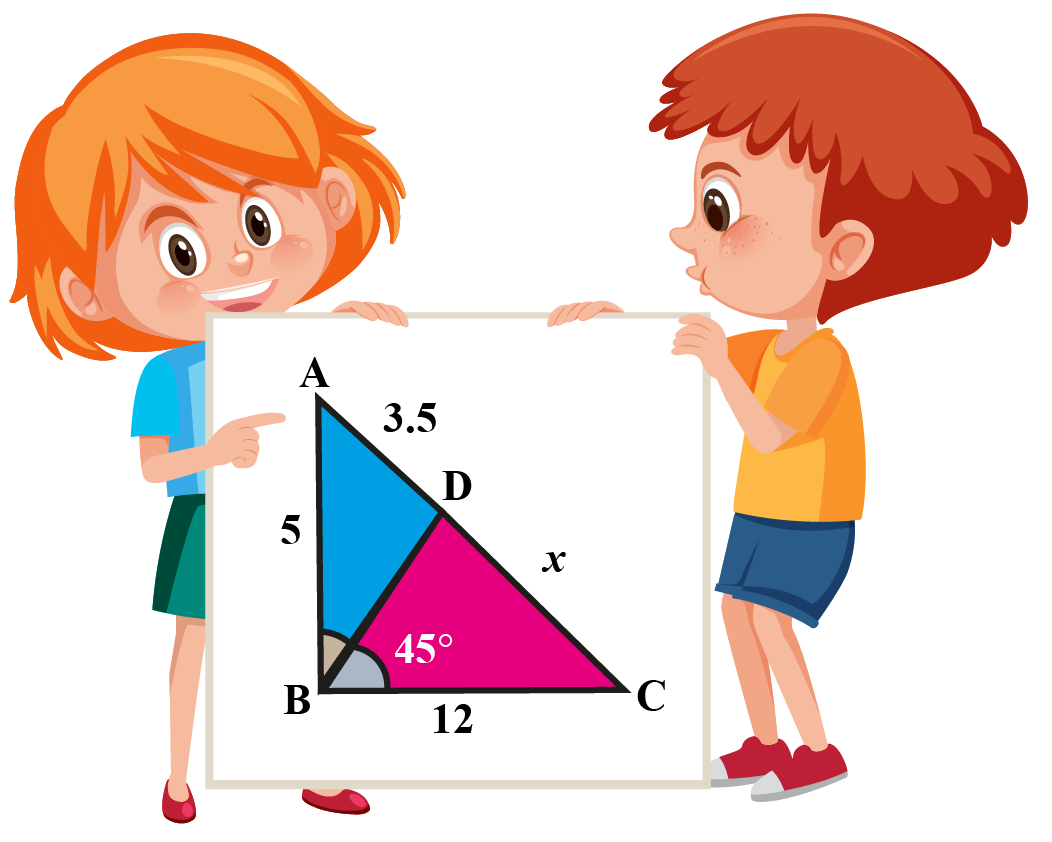She asked Jack to determine the value of $$x$$.

Can you help him?

Solution

In $$\Delta ABC$$, $$\angle DBC=45^{\circ}$$.

Since the triangle is right-angled at $$\angle B$$, we can say that BD bisects the angle $$ABC$$.

By triangle angle bisector theorem, $$\dfrac{AB}{BC}=\dfrac{AD}{DC}$$

Substitute $$AB=5$$, $$BC=12$$, $$AD=3.5$$, and $$DC=x$$.

\begin{align}\dfrac{AB}{BC}&=\dfrac{AD}{DC}\\\dfrac{5}{12}&=\dfrac{3.5}{x}\\5x&=42\\x&=8.4\end{align}

 $$\therefore$$ The length of $$x$$ is 8.4 units.Think Tank
1. Can you use properties of similarity of triangles to prove the triangle angle bisector theorem?
2. Can you find the length of an angle bisector in a triangle? What is the minimum data required to find the length of an angle bisector?

## Interactive Questions

Here are a few activities for you to practice.

Select/type your answer and click the "Check Answer" button to see the result.

## Let's Summarize

We hope you enjoyed learning about the triangle angle bisector theorem with the examples and practice questions. Now, you will be able to easily solve problems on the triangle angle bisector theorem.

## About Cuemath

At Cuemath, our team of math experts is dedicated to making learning fun for our favorite readers, the students!

Through an interactive and engaging learning-teaching-learning approach, the teachers explore all angles of a topic.

Be it worksheets, online classes, doubt sessions, or any other form of relation, it’s the logical thinking and smart learning approach that we, at Cuemath, believe in.

## FAQs on Triangle Angle Bisector Theorem

### 1. What is the formula for angle bisector?

Let AD be the bisector of $$\angle A$$ in $$\Delta ABC$$.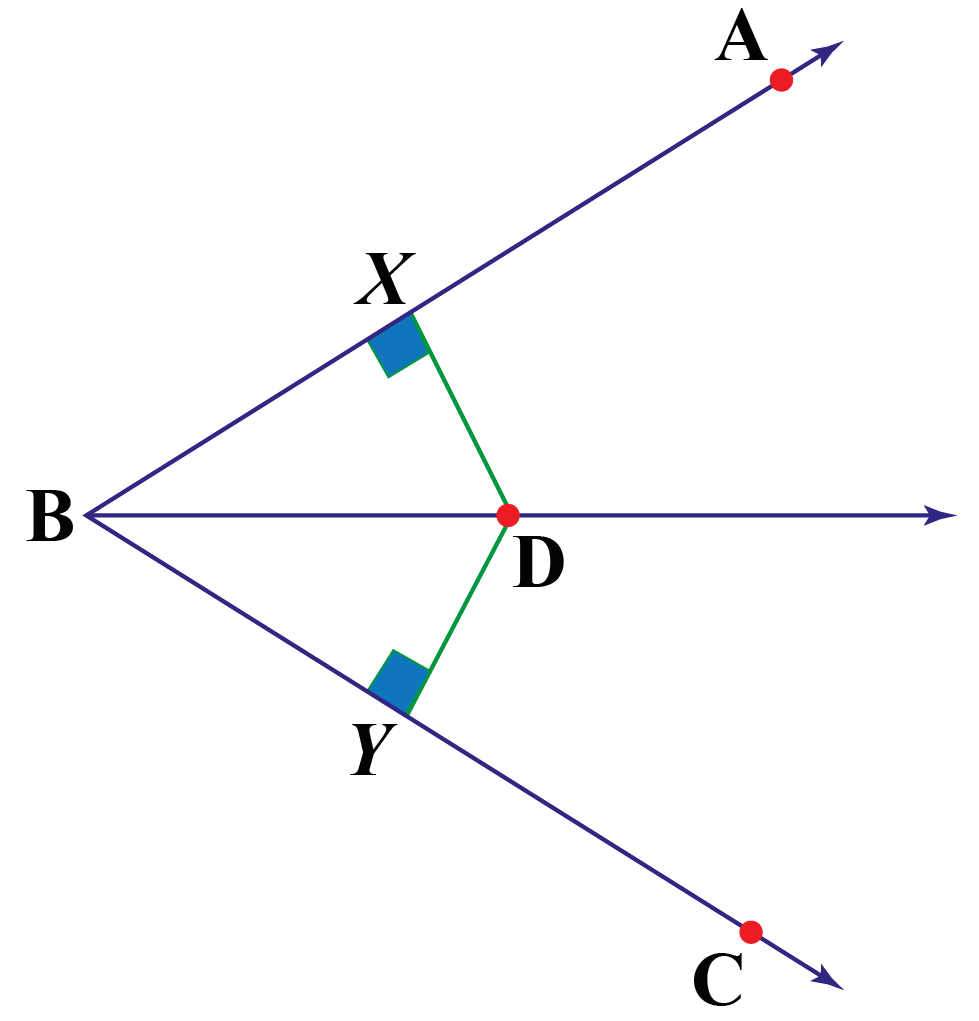According to the angle bisector theorem, $$\dfrac{BD}{DC}=\dfrac{AB}{AC}$$.

### 2. How are the side-splitter theorem and the angle bisector theorem similar?

The only similarity between the side-splitter theorem and the angle bisector theorem is that both the theorems related the proportions of side lengths of the triangle.

### 3. What is the converse of the angle bisector theorem?

If AD is drawn in the $$\Delta ABC$$ such that $$\dfrac{BD}{DC}=\dfrac{AB}{AC}$$, then AD bisects the $$\angle A$$.### 4. How do you find the angle bisector of a triangle?

Follow the steps mentioned below to bisect $$\angle PQR$$.

1. Let Q be the center and with any radius, draw an arc intersecting the ray $$\overrightarrow{QP}$$ and $$\overrightarrow{QR}$$, say at the points E and D respectively.
2. Now, taking D and E as centers and the same radius, draw arcs intersecting each other say at F. <<DE is not a line. Pls check. Also, I guess the radius should be same as the step 1>>
3. Draw the ray $$\overrightarrow{QF}$$.

###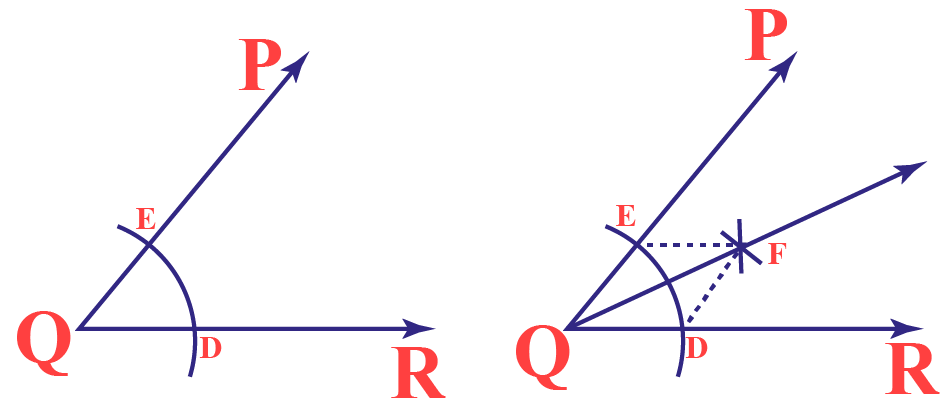Here, $$\overrightarrow{QP}$$ is the angle bisector of $$\angle PQR$$.

### 5. How do you solve a triangle bisector?

We solve a triangle bisector theorem by using the basic proportionality theorem.

### 6. How do you solve an angle bisector problem?

We solve an angle bisector problem by using the triangle angle bisector theorem.

### 7. When can you use the angle bisector theorem?

The angle bisector theorem is used when we know the angle bisector and length of sides of the triangle.

Download Similar Triangles Worksheets
Similar Triangles
grade 9 | Answers Set 1
Similar Triangles
grade 9 | Questions Set 2
Similar Triangles
grade 9 | Answers Set 2
Similar Triangles
grade 9 | Questions Set 1

More Important Topics
Numbers
Algebra
Geometry
Measurement
Money
Data
Trigonometry
Calculus